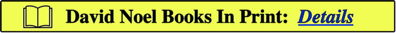BS804: The Q-Scale method of representing masses.

David Noel
<davidn@aoi.com.au>
Ben Franklin Centre for Theoretical Research
PO Box 27, Subiaco, WA 6008, Australia.

Representing very wide ranges of mass
Comparing two masses of objects or classes of objects becomes harder to appreciate or visualize when the ranges of mass included are very large.

For example, comparing the mass of a man with that of a black hole means comparing figures which are many orders of magnitude different. To make such comparisons easier to grasp, a scale of masses has been devised which represents them as the base-10 logarithm of their mass in grams.

This technique for representing very wide ranges may be familiar from the pH Scale used to represent acidity and alkalinity.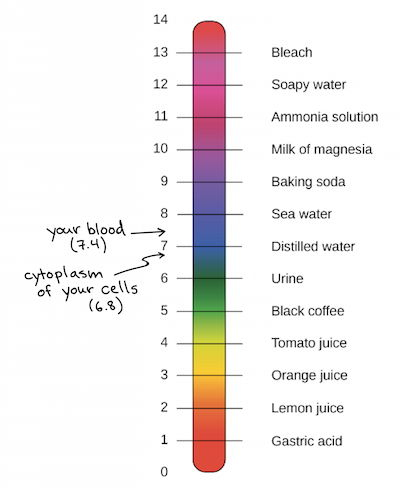Figure BS804-F1. Representing acidity and alkalinity on the pH Scale. From .

In this scale, the pH value or number, which can vary from about 1 up to 14, is actually the negative logarithm of the concentration of Hydrogen ions in a solution. The concentration of hydrogen ions produced by dissociation in pure water is 1 x 10-7 M (moles per litre of water) , or one ten-millionth. So pure water has a pH of 7, the negative of the power of ten.

Moving a pH up or down by 1 means moving the hydrogen ion concentration down or up by a factor of 10, so the pH scale covers a range of concentrations, not of 14 times, but of 1014 times -- a 100 trillion times. Using pH-Scale values makes it easy to grasp the difference between lemon juice (acid) at pH 2 and ammonia solution (alkaline) at pH 11.

The Q-Scale representation of mass
We can represent a very wide scale of masses of objects in terms of their Q-Values (Quantity values), where the Q-Value is the logarithm of the mass in grams.

We can also use any one or more of a number of ranges which cover part of the whole Q-Scale. For example, the masses of various celestial objects may be quoted as multiples of the mass of our Sun, which, according to Google, is 1.989 x 1033 grams.

So the Sun has twice the mass of a Q33 object. Because we are dealing with logarithms, but don't want to get too complicated, we might add just one decimal place, which would put the Sun at Q = 33.3. A star five times the mass of the Sun would have Q = 33.7.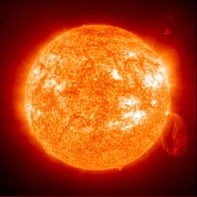Figure BS804-F2. The Sun (Q = 33.3). From .

The masses of stars
Stars are of various masses, from over 100 times that of the Sun, down to 7.5% of the Sun. Very massive stars are exceedingly rare -- the most massive one known is thought to have a mass of 265 Suns.

Ranges of star sizes and properties are shown in the Hertzsprung-Russell star diagram, a nice rendering of which follows.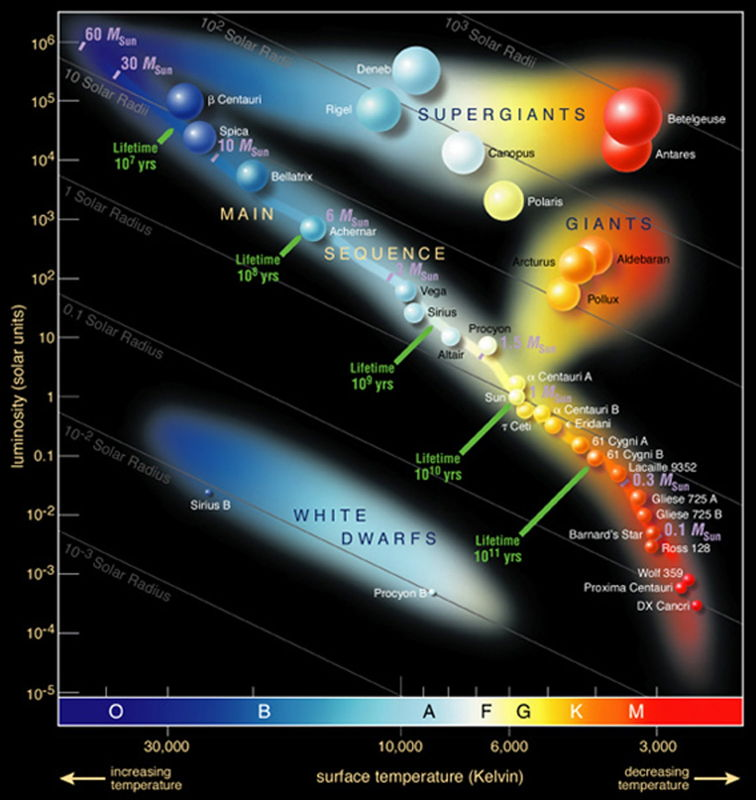Figure BS804-F3. The Hertzsprung-Russell star diagram. From .

This diagram contains a tremendous amount of information, but for the moment we will only focus on star masses. The diagonal bar in Figure 3 (called the "Main Sequence") shows stars arranged from violet giants of highest mass, 60 times that of the Sun, down to red dwarf stars with about 7.5 % of the mass of the Sun. Red dwarfs are exceedingly numerous, the most common type of star.

According to , a star in the Large Magellanic Cloud, at the edge of the Milky Way proper, is the heaviest known star. Called R136a1, it has 265 times the sun's mass. It's classified as a Wolf-Rayet star, and is the most luminous star known, with more than 7 million times the luminosity of our sun. Using the Q-Scale, which has the Sun at Q = 33.3, the heaviest stars would have a Q of around 35, and the lightest stars would be at Q = 32.

The masses of black holes
There are two main types of the objects we call black holes. The first, stellar-mass black holes, are typically in the range of 10 to 100 solar masses (about Q = 34 to 35) . These are the products of the explosion phase encountered by large stars, and so are in the same Q range as such stars.

The second type, supermassive black holes or AGNs (Active Galactic Nuclei), lie at the centres of galaxies, and can be millions or billions of solar masses . The supermassive black hole at the center of the Milky Way, Sagittarius A*, is 4.3 million solar masses. Black holes grow by accreting surrounding matter and by merging with other black holes.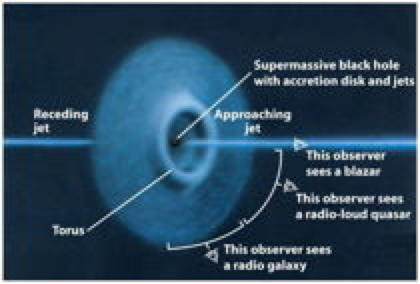Figure BS804-F4. An AGN (Active Galactic Nucleus). From .

At present, the heaviest AGNs are thought to have masses around 10 billion solar masses. So on the Q-Scale, AGNs would have masses of Q = 38 to 43. These are the heaviest "objects" known -- anything heavier, such as a complete galaxy, is a composite entity.

Masses of Earth and the planets
The masses of the planets are often quoted as multiples of the mass of the Earth, 5.972 x 1027 grams -- Q = 27.7 on our scale. Using the figures in NASA's planetary fact sheet , our Solar-System planets have Q-values from about Q = 26.2 (Mercury, the smallest) up to Q = 30.1 (Jupiter, the most massive).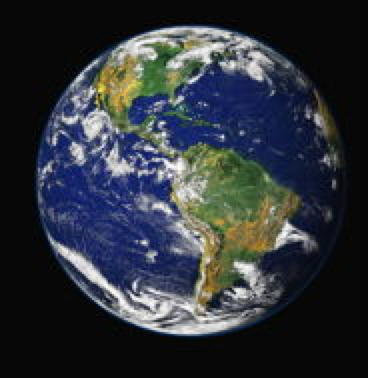Figure BS804-F5. Our planet Earth. From .

All the planets from Earth out have moons, sometimes many -- Jupiter is known to have about 80 -- and these range in mass from bigger than Mercury (for example, Saturn's moon Titan, with Q = 26.3) down to asteroid-size lumps of rock such as Mars' moon Deimos (Q = 18). The Solar System also contains a wealth of asteroids, meteorites, and other debris down to dust-sized particles. There is a comprehensive list at .

This reference  also considers the shapes of moons and asteroids, and says objects more massive than 1021 kilograms are known or expected to be approximately spherical (due to gravity). So it's convenient to put the lower mass limit for planets/moons here, at Q = 24.

In recent years, an increasing number of exoplanets (planets orbiting stars other than the Sun) have been identified. Some of these are as much as 20 times the size of Jupiter , and are "sub-stars" called brown dwarfs -- filling the gap between the largest planets and the smallest stars, at Q = 31.

Masses of living objects
The people around us, from babies to fat adults, have masses of 1 to 400 kg, say Q = 3.0 to 5.2. The largest primate known, now extinct, is thought to have weighed up to 600 Kg.Figure BS804-F6. Extinct primate Gigantopithecus blacki. From .

Elephants clock in at around 5,000 Kg, say Q = 6.7, while Blue Whales can be up to 200 tonnes (Q = 8.3). The lightest known mammal is the Bumblebee Bat, with an adult weighing just 2 grams (Q = 1.3).

When it comes to microscopic creatures, single-celled organisms like amoebas weigh about one-hundredth of a gram (Q = -2). Pollen grains come in at Q = -7 to -9, and a virus cell  (usually reckoned as the smallest living entity) at Q = -15.

Masses of atoms and sub-atomic particles
The Hydrogen atom, the lightest of all atoms, weighs in 1.67 x 10-24 grams , with Q = -23.8. The electron, the lightest elementary particle, has Q = -27. The heaviest atoms, such as lead and uranium, are at Q = -22.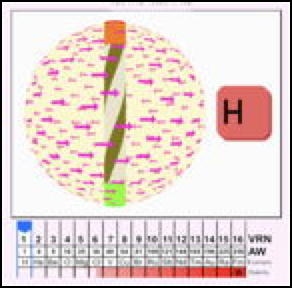Figure BS804-F7. Hydrogen atom in the Spindle-Vortex model. From .

Because Mass and Energy are equivalent, photons of electromagnetic radiation can be regarded as having mass, calculated by the Einstein equation E=mc2. In  it quotes the mass-equivalence of the least-energetic photons known, that of photons at the peak of the spectrum of the cosmic microwave background radiation (CMBR), at 4.2 x 10-37 gm, equal to Q = -36.6.

The Q-Scale graphic
The following graphic gives a simple representation of masses using the Q-Scale, where Q is the base-10 logarithm of an object's mass in grams.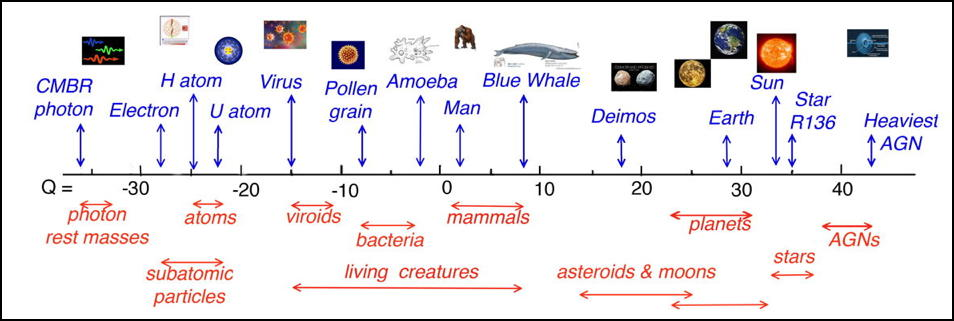Figure BS804-F8. Masses represented on the Q-Scale.

* * * * * * * * * * * * * * * * * *. Acids, bases, pH, and buffers. https://www.khanacademy.org/science/biology/water-acids-and-bases/acids-bases-and-ph/a/acids-bases-ph-and-bufffers .
. Maria Temming. Sizes of Black Holes: How Big is a Black Hole?. https://www.skyandtelescope.com/astronomy-resources/how-big-is-a-black-hole/ .
. The Sun by the Atmospheric Imaging Assembly of NASA's Solar Dynamics Observatory. https://commons.wikimedia.org/wiki/20100819.jpg/ .
. Deborah Byrd. How big are the biggest monster stars?. https://earthsky.org/space/how-big-is-the-biggest-monster-star .
. Hertzsprung-Russell diagram. https://en.wikipedia.org/wiki/Hertzsprung-Russell_diagram .
. List of most massive black holes . https://en.wikipedia.org/wiki/List_of_most_massive_black_holes .
. Age of the Earth. https://simple.wikipedia.org/wiki/Age_of_the_Earth .
. List of Solar System objects by size. https://en.wikipedia.org/wiki/List_of_Solar_System_objects_by_size .
. List of largest exoplanets. https://en.wikipedia.org/wiki/List_of_largest_exoplanets .
. Gigantopithecus blacki. https://www.deviantart.com/kaek/art/Gigantopithecus-blacki-600210095.
. Orders of magnitude (mass). https://en.wikipedia.org/wiki/Orders_of_magnitude_(mass) .
. David Noel. BS802: GEMMA -- The Spindle Vortex Model for Gravity, Energy, Matter, Magnetism, Antimatter. http://aoi.com.au/BaseScience/BS802-Vortex/ .